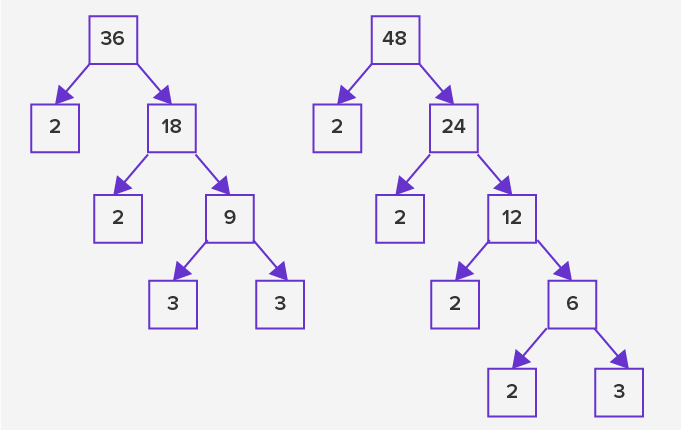# Least Common Multiple - Definition with Examples

The Complete K-5 Math Learning Program Built for Your Child

• 40 Million Kids

Loved by kids and parent worldwide

• 50,000 Schools

Trusted by teachers across schools

• Comprehensive Curriculum

Aligned to Common Core

## Least Common Multiple

Multiple is a number that can be divided by the given number without leaving a reminder. For example:

20 is a multiple of 5

Or, 5 × 4 = 20

And, 20 ÷ 5 = 4

## Least common multiple

The least common multiple of two numbers is the “smallest non-zero common number” which is a multiple of both the numbers.

The different methods to find least common multiple of two or more numbers are:

• Using prime factorization
• Using repeated division
• Using multiples

1. LCM using prime factorization

In this method, a factorization tree for each given number is generated by listing the multiples of that number. The last branch of the tree has the least prime factors for that number.

For example, the factorization trees for 36 and 48 are generated as follows:Figure: Prime factorization trees for the number 36 and 48

To find the LCM, pair the common multiples as shown. List them along with the remaining multiples.LCM = 2 × 2 × 3 × 3 × 2 × 2

LCM = 144

2. LCM using repeated division

In this method, the given numbers are divided by the common divisors until there is no possible further division by the common number. The divisors and the remainders are multiplied together to obtain the LCM.LCM =  2 × 2 × 3 × 4 × 3

LCM = 144

3. LCM using multiples

To find the LCM using multiples, list the multiples of the numbers in the table as shown. The least common multiple is the first common multiple for the given numbers.

 1 2 3 4 5 6 7 8 9 10 11 12 36 36 72 108 144 180 216 252 288 324 360 396 432 48 48 96 144 192 240 288 336 384 432 480 528 576

For 36 and 48, the number 144 is the LCM.

## Application

The dimension of using LCM of two numbers starts with basic math operations such as addition and subtraction on fractional numbers. In math problems where we pair two objects against each other, the LCM value is useful in optimizing the quantities of the given objects. Also, in computer science, the LCM of numbers helps design encoded messages using cryptography.

 Fun facts Greeks used the wax tablets to record multiplication tables in 1st century AD William Oughtred used the symbol “?” for multiplication in the 15th century to teach math

Related math vocabulary

• Multiples and multiplication
• Highest Common Factor (HCF)
• Fractions
Won Numerous Awards & Honors